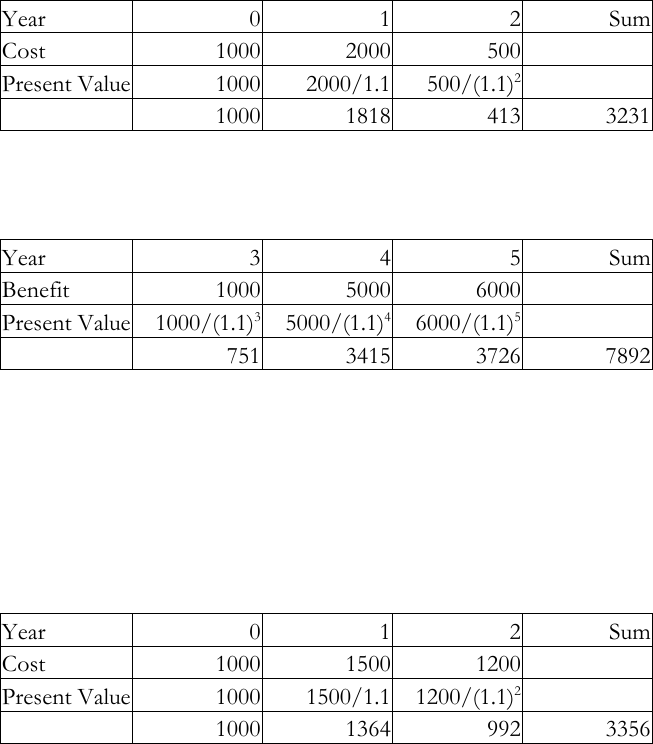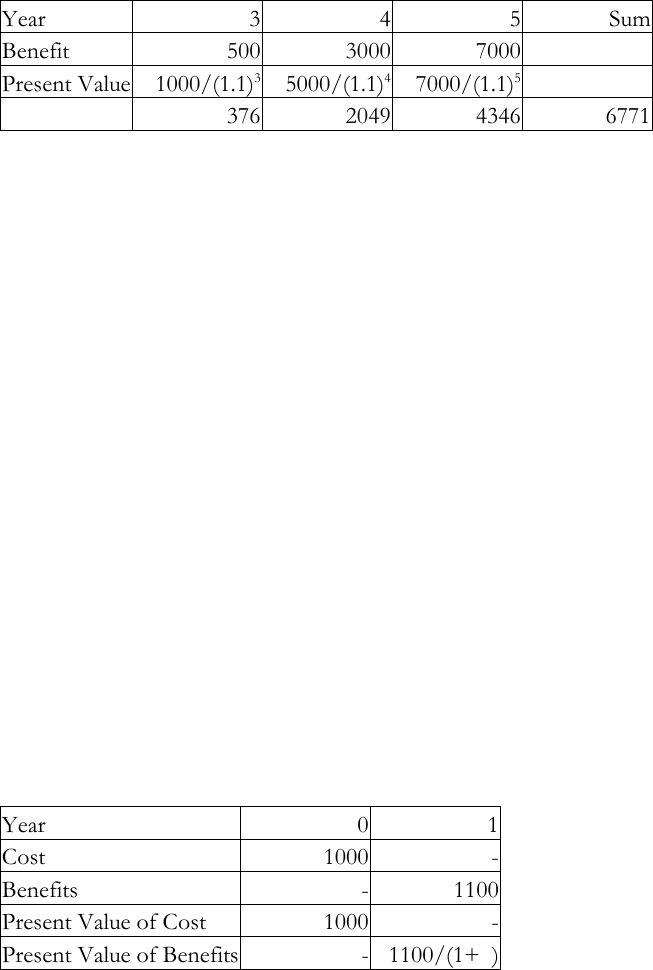# ECON 3650 Lecture Notes - Lecture 5: Federal Aviation Administration, National Highway Traffic Safety Administration, United States Treasury Security

31 views11 pages1
ECON 3650: Policy Analysis
Lecture 5 Tools of Policy Analysis
Financial Accounting Analysis and Cost Benefit Analysis
Resources: Chapter 8 : Jonathan Gruber
In many cases of policy analysis, current and future costs of policies are to be compared
against current and future benefits. Financial accounting analysis and cost-benefit analysis
are two major tools of analysis.
5.1 Financial Accounting Analysis
Time Value of Money
Example 1:
i. A \$100 received next year (year 1) is worth less than \$100 this year (year 0).
This is because money loses its value over time.
The discount factor = 1/(1+market interest rate) interest rate = price of money
If the market interest rate is 5%, then the discount rate = 1/(1+5/100) = 0.95
a. \$100 to be received in 2021 is worth = 100/(1+5%) = 100*0.95 = \$95
b. \$100 to be received in 2022 is worth = 100/(1+5%)2 = 100*.95*.95 = 90.25
ii. A \$100 received in this year (year 0) is worth more than \$100 next year (year 1).
This is because value of money is greater this year than next year.
The compounding factor = (1+5%) = 1.05
a. Thus, \$100 received in 2020 is worth 100*(1.05) = \$105
Money can’t just be added together over time because it is discounted
Example 2
Use the time value of money concept to evaluate these two projects
Project 1:
Cost - 1000 in year 0, 2000 in year 1 and 500 in year 2
Unlock document

This preview shows pages 1-3 of the document.
Unlock all 11 pages and 3 million more documents.2
Benefits - 1000 in year 3, 5000 in year 4, and 6000 in year 5.
Project 2 :
Cost - 1000 in year 0, 1500 in year 1 and 1200 in year 2
Benefits - 500 in year 3, 3000 in year 4, and 7000 in year 5.
Pure Financial Analysis:
Use the market interest rate to discount future stream of costs and benefits.
Choose the project with highest net benefits.
(Market interest rate say 10%)
Discount factor = 1/(1+10%) = 1/1.1 = 0.91
Project 1
Present Value of Costs of Project 1
Year
0
1
2
Sum
Cost
1000
2000
500
Present Value
1000
2000/1.1
500/(1.1)2
1000
1818
413
3231
Present Value of Benefits of Project 1
Year
3
4
5
Sum
Benefit
1000
5000
6000
Present Value
1000/(1.1)3
5000/(1.1)4
6000/(1.1)5
751
3415
3726
7892
Net Present Value from Project 1 = 7892-3231 = USD 4661
Project 2
Present Value of Costs of Project 2
Year
0
1
2
Sum
Cost
1000
1500
1200
Present Value
1000
1500/1.1
1200/(1.1)2
1000
1364
992
3356
Unlock document

This preview shows pages 1-3 of the document.
Unlock all 11 pages and 3 million more documents.3
Present Value of Benefits of Project 2
Year
3
4
5
Sum
Benefit
500
3000
7000
Present Value
1000/(1.1)3
5000/(1.1)4
7000/(1.1)5
376
2049
4346
6771
Net Present Value from Project 1 = 6771-3356 = USD 3415
Based on Financial Analysis Project 1 is better.
5.2 Internal Rate of Return = when net percent value becomes 0
Internal rate of return of a project is the rate of interest at which the net present value
becomes zero.
Example 3
If you invest \$1000 in a project that lasts just one year, and receives \$1100 in return at the
end of the year the internal rate of return is 10%.
The above figure was achieved by using the following procedure.
Assume that the internal rate of return is x.
The cash flow of the project is as follows.
Year
0
1
Cost
1000
-
Benefits
-
1100
Present Value of Cost
1000
-
Present Value of Benefits
-
1100/(1+x)
Net Present Value = 1100/(1+x) 1000
Net Present Value is zero when x=10%
Example 4
Unlock document

This preview shows pages 1-3 of the document.
Unlock all 11 pages and 3 million more documents.